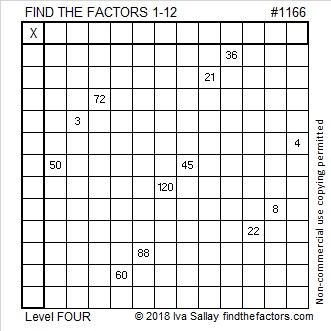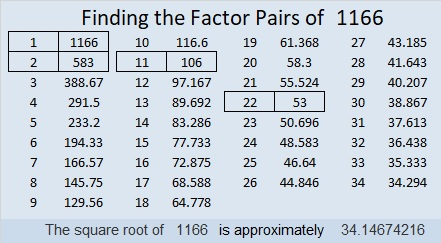# 1166 and Level 4

Study the clues in this puzzle. Find the most logical place to start and begin there. Once you find all the factors you will see how amazing YOU are! You can do this!Print the puzzles or type the solution in this excel file: 12 factors 1161-1173

Now I’ll share some information about the number 1166:

• 1166 is a composite number.
• Prime factorization: 1166 = 2 × 11 × 53
• The exponents in the prime factorization are 1, 1, and 1. Adding one to each and multiplying we get (1 + 1)(1 + 1)(1 + 1) = 2 × 2 × 2 = 8. Therefore 1166 has exactly 8 factors.
• Factors of 1166: 1, 2, 11, 22, 53, 106, 583, 1166
• Factor pairs: 1166 = 1 × 1166, 2 × 583, 11 × 106, or 22 × 53
• 1166 has no square factors that allow its square root to be simplified. √1166 ≈ 34.146741166 is the hypotenuse of a Pythagorean triple:
616-990-1166 which is 22 times (28-45-53)

This site uses Akismet to reduce spam. Learn how your comment data is processed.